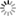##### Personal tools
You are here: Home Mathematics for Business and Finance

# Mathematics for Business and Finance

Business mathematics is the mathematics used by business enterprises to record and manage business operations. Business organizations use mathematics in accounting, inventory management, marketing, sales forecasting, and financial analysis.

Mathematics commonly used in business includes elementary arithmetic, elementary algebra, statistics, and probability. For some management problems, more advanced mathematics is applied - such as calculus, matrix algebra and linear programming.

### - Mathematical Finance

Mathematical finance, also known as quantitative finance and financial mathematics, is a field of applied mathematics that focuses on the mathematical modeling of financial markets.

Generally speaking, there are two separate branches of finance that require advanced quantitative techniques: derivatives pricing on the one hand, and risk and portfolio management on the other.

Mathematical finance overlaps considerably with the fields of computational finance and financial engineering. The latter focuses on applications and modeling, often with the help of stochastic asset models, while the former focuses on building implementation tools for models in addition to analysis. Also relevant is quantitative investing, which relies on statistical and numerical models (and more recently machine learning) rather than traditional fundamental analysis when managing portfolios.

• Behavioral finance
• Commodity markets
• Computational finance
• Credit risk
• Intelligent and automated Asset management
• Insurance mathematics
• Market microstructure
• Market liquidity and frictions
• Mean field games
• Model Risk and Uncertainty
• Optimal stopping
• Optimal transport
• Option pricing and hedging
• Risk measures
• Robust finance
• Stochastic control
• Stochastic games
• Stochastic volatility
• Systemic risk
• Stochastic modeling of epidemics[Amsterdam, Netherlands - Civil Engineering Discoveries]

### - Computational Mathematics

Computational mathematics involves the study of mathematics in the fields of mathematics and science, where computation plays a central and important role, and emphasizes algorithms, numerical methods, and symbolic computation.

Computational applied mathematics broadly includes the use of mathematics in applied mathematics to allow and improve computer computation. Computational mathematics can also refer to the use of computers for mathematics itself. This includes using computers to perform mathematical calculations (computer algebra), studying what in mathematics can (and cannot) be computerized (efficient methods), which calculations can be done with existing technology (complexity theory), and which proofs can be done on a computer (Proof Assistant).

• Statistical Computing
• Mathematical Modeling
• Applied Mathematics
• Computational Mathematics
• Financial mathematics
• Hedge funds and asset-market dynamics
• Hedging strategies
• Arbitrage techniques
• Rational expectation models
• Game theory
• Risk management and systemic risk
• Risk capital and capital allocation
• Financial econometrics
• Actuarial science
• Empirical finance
• Leverage ratios
• Asset pricing
• Risk management
• Risk assessment and analysis
• Securitization
• Primary and derivative securities valuation
• Term structure models
• Term structure of interest rate
• Interest rate modeling
• Investment management
• Portfolio optimization and management
• Portfolio models
• Private equity
• Swaps & derivatives trading or dealing
• Derivative markets
• Stocks and exchange rates prediction
• Insurance pricing and modeling
• Distribution of insurance risk
• Bankruptancy prediction
• Financial & stock market monitoring and prediction
• Credit assessment
• Credit fraud detection
• Money laundering surveillance
• Bank capital adequacy, stress testing, market liquidity risk
• Fintech (financial technology): emerging financial services
• Bitcoin and blockchain technology

[More to come ...]

##### Document Actions